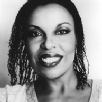# Let It Be Keyboard

## Roberta Flack

Difficulty:Easy

by ARICAL## Let It Be

( John Lennon and Paul McCartney)

Key:  CLet It Be Key A#A#(one step down)
Let It Be Key BB(half step down)
Let It Be Key CC(original key)
Let It Be Key C#C#(half step up)
Let It Be Key DD(one step up)
```
C                     G                        Am                   F
When I find myself in times of trouble, Mother Mary comes to me
C                                 G                F  C   Dm   C
Speaking words of wisdom, let it be
C                          G                  Am                           F
And in my hour of darkness, she is standing right in front of me
C                                   G              F  C  Dm   C
Speaking words of wisdom, Let it be
G     Am              G               F             C
Let it be,      let it be,     let it be,     let it be
G                 F  C  Dm  C
Whisper words of wisdom, let it be
C              G                 Am          F
And when the broken hearted people, Living in the world agree
C                 G              F  C   Dm   C
There will be an answer, let it be
C              G                 Am          F
But though they may be parted, There is still a chance that they may see
C                 G              F  C/E   Dm   C
There will be an answer, let it be
Am            G              F            C
Let it be, let it be,   let it be,    let it be
G                C              F  C/E   Dm    C
There will be an answer, let it be
G     Am              G               F             C
Let it be,      let it be,     let it be,     let it be
G                 F  C  Dm  C
Whisper words of wisdom, let it be
G     Am              G               F             C
Let it be,      let it be,     let it be,     let it be
G                 F  C  Dm  C
Whisper words of wisdom, let it be
C          G                      Am     F
And when the night is cloudy, there is still a light that shines on me
C                       G                  F  C   Dm   C
Shine on till tomorrow, let it be
C                             G                 Am                          F
I wake up to the sound      of music, Mother Mary comes    to me
C                                 G                 F  C  Dm   C
Speaking words of wisdom, let it be
G     Am              G               F             C
Let it be,      let it be,     let it be,     let it be
G                 F  C  Dm  C
Whisper words of wisdom, let it be
G     Am              G               F             C
Let it be,      let it be,     let it be,     let it be
G                 F  C  Dm  C
Whisper words of wisdom, let it be
```Full key step upHalf key step upHalf key step downFull key step downshow chordsYouTube Cliphide all tabsgo to top